# Davor JosipovicJust another WordPress blog – rather tryout

## 18/08/2017

### Calvin’s optimal way home

Filed under: Algorithms,Modeling,Statistics — Tags: , — Davor @ 18:28

This puzzle has been posted here previously but with no correct answer, except for (a). It has also been used by Optiver for the assessment of their new quantitative researchers.

Calvin has to cross several signals when he walks from his home to school. Each of these signals operate independently. They alternate every 80 seconds between green light and red light. At each signal, there is a counter display that tells him how long it will be before the current signal light changes. Calvin has a magic wand which lets him turn a signal from red to green instantaneously. However, this wand comes with limited battery life, so he can use it only for a specified number of times.

a. If the total number of signals is 2 and Calvin can use his magic wand only once, then what is the expected waiting time at the signals when Calvin optimally walks from his home to school?
b. What if the number of signals is 3 and Calvin can use his magic wand only once?
c. Can you write a code that takes as inputs the number of signals and the number of times Calvin can use his magic wand, and outputs the expected waiting time?

## Solution

My solution to all three questions can be found here: Davor, puzzle solution, 2017. I think it can be interesting to people who have never done statistical modeling and would like to know how it is done.

a. Expected trip time is 8.75 sec. Optimally, wand should be used on red light if the counter is above 20 sec.
b. Expected trip time is 21.32 sec. Optimal wand usage at first light is if the counter is above 31.25 sec, and 20 at the second if there is a charge left.
c. See the solution for the recursive 10-line code that solves the general case. For example, if Calvin has 2 charges and there are 4 lights, then the expected trip time is 11.8 sec with the optimal wand usage.

## 04/07/2017

### Extending Berman’s ICE algorithm with spatial information

Filed under: Algorithms,Modeling,Statistics — Tags: , — Davor @ 16:08
Note: this article is a short summary of a larger work I have done here.

## Short introduction

Hyperspectral images are like ordinary images, except that they have lots of bands extended beyond the visible spectrum. This extra information is exploited for material identification. For example, in this hyperspectral image a sub-scene is selected – called the Alunite Hill.The original Cuprite scene from which a 16 x 28 pixel subimage is extracted for analysis.

Subsequently, the ICE algorithm is run which results in the following three material abundance maps contained in matrix$\mathbf{W}$ and material signatures$\mathbf{E}$ called endmembers.Output of the ICE algorithm: abundance maps for alunite, muscovite and kaolinite minerals, and their hyperspectral signatures, i.e. endmembers.

## ICE algorithm

Simplified, the ICE algorithm can be written as an objective function$L_{ICE}$ which measures the error between the actual pixels and the predicted pixels$||\mathbf{X} - \mathbf{W}\mathbf{E}^T ||_F^2$ together with a regularization term$V(\mathbf{E})$ that measures the size of the simplex formed by the endmembers.$L_{ICE}(\mathbf{E}, \mathbf{W}) = ||\mathbf{X} - \mathbf{W}\mathbf{E}^T ||_F^2 + V(\mathbf{E})$

This objective function$L_{ICE}$ is subsequently minimized to get the estimates of the abundance maps and endmembers:$\underset{\mathbf{E}, \mathbf{W}}{argmin} \; L_{ICE}(\mathbf{E}, \mathbf{W})$

## Spatial information

The idea that spatial information is important stems from the fact that materials in abundance maps are more likely to reflect certain order.Two abundance maps of the same material. The pixels are identical, but randomly ordered in the right one.

Thus the right abundance map in which the material seems randomly scattered, should be penalized more than the left one where there seems to be a certain order. One way to achieve this is by looking at the adjacent pixels of the abundance maps and see how similar they are. In this specific approach we are calculating the variance of a pixel and its adjacent four pixels. These variances are summed over all pixels of the abundance maps:$S(\mathbf{W}) = \sum_n \sum_m \text{Var}(\mathbf{u}_{n,m})$

where$\mathbf{u}_{n,m}$ signifies the vector of the abundance and the adjacent abundances of the$n$-th pixel and$m$-th endmember. The new simplified objective functions becomes$L_{ICE-S}(\mathbf{E}, \mathbf{W}) = ||\mathbf{X} - \mathbf{W}\mathbf{E}^T ||_F^2 + V(\mathbf{E}) + S(\mathbf{W})$

This new objective function is then minimized:$\underset{\mathbf{E}, \mathbf{W}}{argmin} \; L_{ICE-S}(\mathbf{E}, \mathbf{W})$

The abundance maps resulting from the minimization of$L_{ICE-S}$ tend to be more smooth.Output of ICE-S algorithm. The abundance maps are slightly more smooth when compared with the ICE output above. The central pixel in the third abundance map seems to be completely removed.

## Conclusion

My main aspiration here was to give a very concise and simplified version of how the ICE algorithm can be extended with spatial information. The actual topic is much more complex. For those interested: The theoretical foundations, calculus and implementation details can be found here.

## 02/02/2017

### Primal Active-Set method for constrained convex quadratic problem

Filed under: Algorithms — Tags: , — Davor @ 10:05

I made an implementation of the Active-Set Method for Convex QP as described in Nocedal, J. e.a., Numerical Optimization, 2ed, 2006, p.472. The code in R can be found on Github. Output with two examples from the book can be found here.

There is an other free package named quadprog that does the same but with the limitation of only accepting positive definite matrices. This can be tweaked to work with positive semi-definite matrices. For example one can convert a positive semi-definite matrix to its nearest definite one with Matrix::nearPD() function. To cope with semi-definiteness in the Active-Set method, Moore-Penrose generalized inverse is used for solving the KKT problem.

Note that the Active-Set method must start with an initial feasible point. Understanding the problem is usually enough to calculate one. Nocedal describes a generic “Phase I” approach for finding a feasible point on p.473.

## 28/11/2016

### What is so semantic in Semantic web anyway?

Filed under: Philosophy — Tags: , — Davor @ 11:09

Semantic web. Semantic? This eluded me back from the time I first heard the term. It is concerned with the meaning and not as much with the structure of data. But how?

The term “semantic” was coined by Tim Berners-Lee for a web of data that can be processed by machines. But that doesn’t tell us why it is called semantic. What has meaning to do with machines and processing?

After I saw this MIT presentation on the suject it dawned upon me that there is an interesting equivalence between how truth is defined in Semantic web, and the way Donald Davidson defined meaning in his “theory of meaning”. His ideas go back to the 60s, and are based on Tarski’s theory of truth from the 30s. So here is my colloquized way of explaining the intuition of why the Semantic web is actually semantic.

The semantinc web in the MIT presentation is defined as:

XML + RDF + Ontologies + Inference rules = Semantic web!

You wonder why all this equates to “Semantic”?

Suppose you have a set of entities, lets say {Socrates, man, mortal}. Suppose don’t know about nothing else than those three words. Suppose that that is your Ontology. That is your world. Note that having an Ontology is a constitutive requirement for a Semantic web. So are the Inference rules. Suppose we have only one Inference rule: if A is B, and B implies C then A is C. The third constitutive component (RDF) consists of  some true statements about your set of objects which are contained in the subjectpredicateobject structure. For example: Socrates (subject) is (predicate) a man (object) and men (subject) are (predicate) mortal (object). XML is used to describe all of the other three so a machine can read and interpret them. Now given the preceding example, what the machine can do is deduce the truth of a statement like: Socrates is a man, all men are mortal, therefore Socrates is mortal (implication from Inference rule). Now remember (!), given its Ontology, Inference rules and RDF, the machine knows which statement is true, and which is not true. The machine knows that the statement Socrates is mortal is true!

Now, where is semantics in all this? Well, Davidson proposed the idea that truth and meaning are equivalent. If you have one, then you have the other too. Note that given the RDFs, Ontology and Inference rules, the machine actually knows when any statement is true. Thus the machine knows the meaning of the statement.

That too quick? Not convinced? Well, suppose I ask you whether the following statement is true: “Socrates je covjek”. Can you assess the truth? Not if you don’t know Croatian – which I assume you do not for the sake of the example. But suppose I tell you that the statement “Socrates je covjek” is true under all conditions under which “Socrates is a man” is true (i.e. the two statements are equivalent). Since you know that “Socrates is a man” is true (i.e. it is explicitly stated in your RDF), and that “Socrates je covjek” is true whenever “Socrates is a man” is true, then you can perfectly say that you understand “Socrates je covjek” and thus know its meaning. In other words, if you know the truth conditions of “Socrates je covjek”, you understand it. The same can be done with “Socrates is mortal”. Although it is not explicitly stated in the RDF, the machine can deduce its truth. So if “Chapa muju koki” is equivalent to “Socrates in mortal”, then you can say that you understand it. That is the mechanism behind the Semantic web. Knowing the truth of a statement implies knowing the meaning of the statement – and vice versa.

Thus the web is semantic.

Now you can ask whether meaning is not more than only truth. That is a difficult question for which I can not go into much details here. My philosophical days are unfortunately numbered. But a good starting point on this subject is probably here. My own intuition is that meaning as defined above is only a subset of what we understand under meaning. Thus, the web is semantic, but only up to a certain degree…

## 30/08/2014

### Transform any (binary) function to an aggregate in pure SQL

Filed under: Oracle — Tags: , — Davor @ 10:44

Few days back I needed an aggregate counterpart of a BITOR function. Unfortunately the Oracle database doesn’t have one.

So how do I make one? There are three options here:

1. Write your own aggregate function. Here is one way to do it.
2. Rewrite it in function of an other aggregate function. For example PRODUCT() can be rewritten as EXP(SUM(LN())) (cf. infra). But there is no obvious way for writing an aggregate BITOR() in function of existing Oracle functions.
3. Simulate aggregation in pure SQL. If you for example have 4 elements {a, b, c, d}, you know that their BITOR aggregate is: BITOR(a,BITOR(b,BITOR(c,d))). Since SQL:1999 we have recursion in SQL. So why write an aggregate function if you can compute it within SQL?

This blogpost is all about the third option. I wanted to see (I) whether it is possible and (II) whether I can generalize it in a concise manner for all binary functions. I also couldn’t find any information about this on the Internet, so that is why I am writing this. It turns out (I) is true, and (II) also, albeit with complications. What I didn’t expect is very bad performance. So the solution below is only for educational use.

### Aggregation with + operator

Let’s start with summation. Summation (+) is a binary operator/function that is easy to understand and easy to verify with the aggregate SUM() function.

CREATE TABLE t4 ( gr NUMBER(10), -- group nr NUMBER(10) -- number );

Insert some values in it

INSERT INTO t4 VALUES (1,10); INSERT INTO t4 VALUES (1,20); INSERT INTO t4 VALUES (1,30); INSERT INTO t4 VALUES (1,40); INSERT INTO t4 VALUES (1,50); INSERT INTO t4 VALUES (2,20); INSERT INTO t4 VALUES (2,30);

What we have is this:

        GR         NR
---------- ----------
1         10
1         20
1         30
1         40
1         50
2         20
2         30


The way to sum-aggregate these numbers is by adding a new column which will compute the sum of the current number and the previous sum:

        GR         NR RECURSIVE_SUM
---------- ---------- -------------
1         10            10
1         20            30
1         30            60
1         40           100
1         50           150
2         20            20
2         30            50


Finally I just have to take the last step in each group: 150 for gr = 1 and 50 for gr = 2. The recursive query that does all the above and uses only the (+) operator is the following:

WITH sel AS ( SELECT t4.nr, t4.gr, ROW_NUMBER() OVER (partition BY gr ORDER BY nr) AS rn FROM t4 ), rec(gr, r_out, rn, nr) AS ( SELECT gr, nr, rn, nr FROM sel WHERE rn = 1 UNION ALL SELECT sel.gr, rec.r_out + sel.nr, rec.rn + 1, sel.nr FROM rec, sel WHERE sel.rn = rec.rn + 1 AND sel.gr = rec.gr ), gr AS ( SELECT gr, COUNT(gr) AS MAX FROM sel GROUP BY gr ) SELECT gr.gr, r_out SUM FROM gr INNER JOIN rec ON (gr.max = rec.rn AND gr.gr = rec.gr) ORDER BY gr.gr;
        GR        SUM
---------- ----------
1        150
2         50



This is equivalent to:

SELECT SUM(nr) SUM FROM t4 GROUP BY gr ORDER BY gr;
        GR        SUM
---------- ----------
1        150
2         50



### Aggregation with * operator

Now let’s try multiplication. Just like summation, a product (*) is a binary operator/function. Now, to simulate the PRODUCT() function with the binary * function, you only have to change the r_out field in the recursive query:

WITH sel AS ( SELECT t4.nr, t4.gr, ROW_NUMBER() OVER (partition BY gr ORDER BY nr) AS rn FROM t4 ), rec(gr, r_out, rn, nr) AS ( SELECT gr, nr, rn, nr FROM sel WHERE rn = 1 UNION ALL SELECT sel.gr, rec.r_out * sel.nr, rec.rn + 1, sel.nr FROM rec, sel WHERE sel.rn = rec.rn + 1 AND sel.gr = rec.gr ), gr AS ( SELECT gr, COUNT(gr) AS MAX FROM sel GROUP BY gr ) SELECT gr.gr, r_out product FROM gr INNER JOIN rec ON (gr.max = rec.rn AND gr.gr = rec.gr) ORDER BY gr.gr;
        GR    PRODUCT
---------- ----------
1   12000000
2        600


We can verify the above outcome with the aggregate PRODUCT() function rewritten as EXP(SUM(LN())). With a little algebra you can figure out why the equation holds. Here is the statement:

SELECT gr, EXP(SUM(LN(nr))) AS product FROM t4 GROUP BY gr ORDER BY gr;
        GR    PRODUCT
---------- ----------
1   12000000
2        600


### Aggregation with BITOR function

By now it should be clear how to adjust the recursive query to simulate aggregation for any binary function: We only have to adjust the r_out field. Now let’s try to simulate the aggregated BITOR function. Because there is no BITOR we can rewrite it as BITOR(x,y) = (x+y)-BITAND(x,y);

WITH sel AS ( SELECT t4.nr, t4.gr, ROW_NUMBER() OVER (partition BY gr ORDER BY nr) AS rn FROM t4 ), rec(gr, r_out, rn, nr) AS ( SELECT gr, nr, rn, nr FROM sel WHERE rn = 1 UNION ALL SELECT sel.gr, (rec.r_out + sel.nr) - BITAND(rec.r_out, sel.nr), rec.rn + 1, sel.nr FROM rec, sel WHERE sel.rn = rec.rn + 1 AND sel.gr = rec.gr ), gr AS ( SELECT gr, COUNT(gr) AS MAX FROM sel GROUP BY gr ) SELECT gr.gr, r_out BITOR FROM gr INNER JOIN rec ON (gr.max = rec.rn AND gr.gr = rec.gr) ORDER BY gr.gr;
        GR      BITOR
---------- ----------
1         62
2         30


Note 1: all the above recursive code is very inefficient. Tables exceeding 100 values will have a large performance impact. The problem is the statement following the UNION ALL: it has to select the right value(s) for each recursive iteration step. When I find more time I’ll try to optimize it. In the meantime, here is a query to fill the table with some dummy values for testing:

INSERT INTO t4 SELECT round(dbms_random.value(1,5)), round(dbms_random.value(1,99)) FROM DUAL CONNECT BY level <= 100;

## 16/08/2013

Filed under: PowerShell,Programming — Tags: , — Davor @ 15:36

I don’t like the fact that there are so many backup programs, most of which are expensive closed source solutions. All have their own (mostly) undocumented and proprietary archive systems, that are hardly usable without the software that made them. All this… while Windows 7 offers enough technology under the hood to make an open source scripted solution possible, where the files are versioned, incremental and hardlinked (thus saving space), while the versioned backup contents can be viewed in Explorer. No extra software is needed. The current version of the script you can find on GitHub.

Here is an overview:Root of the backup folder. The contents are hardlinked, which means that 10 identical files backed up at different times take only the space of 1 file.

The includelist file for the backup might look like this:

D:\Hardware\* M:\Music\Playlists\* M:\Pictures\* W:\Research\* W:\Server\apache\*\*.conf

And this is an example of how to run the backup script:

.\ps-backup.ps1 -Backup -BackupRoot "W:\Backups\Archive" -SourcePath "W:\Scripts\ps-backup\include_list.txt" -ExclusionList "W:\Scripts\ps-backup\exclude_list.txt"

## 02/06/2013

### Ignoring device I/O errors during copy with PowerShell

Filed under: PowerShell,Programming — Tags: , , — Davor @ 19:14

I had a failing hard drive with lots of RAR files with recovery records. Problem was that I couldn’t get the files off the hard drive with tools like robocopy and xcopy because the drive had many bad sectors resulting in CRC and I/O device errors. I also couldn’t repair the drive with chkdsk /R because the bad sectors kept reappearing.

I also tried Unstoppable Copier, but on best settings it seems to use 0,1% of the file size per read operation, which results in large data corruption when that read operation fails even if there are only a few bad sectors.

So I wrote a little PowerShell script which will copy the source files and replace the unreadable data with zero’s, as accurately as the partition’s cluster size allows. It will also make a XML file per file where it will store bad sector data positions for further reference. I have placed the script code on GitHub.

When a hard drives fails to read a sector due to a CRC error, it doesn’t give back any data. Instead it raises an error: I/O device error. With old hard drives it was possible to issue the READ LONG command to skip the error correction part, and simply give back the data. Some programs like Spinrite use this to recover data based on re-read statistics. (If on hundred consecutive re-read operations for sector X your starting bits are “1011…”, then it is reasonably safe to assume those bits are not corrupted.) But the READ LONG command doesn’t work with modern hard drives. Modern hard drives either succeed or fail a read operation. There is no third option. What I have noticed during my own recovery is that even though the hard drive fails reading a sector the first time, there is some chance it will succeed on a retry. I have seen it succeed even after 100 fails! That’s why the -MaxRetries is so important in this script. But setting it too high will greatly slow down the recovery process in case a sector is truly unreadable.

## Notes on script usage

1. First you need to download the script. Here is a link to the file. Save it as Force-Copy.ps1 to, for example, your desktop. (Make sure the extension is correct: ps1!) (There is also a more simple script available at stackoverflow.)
2. This is a PowerShell script, so it has to run under the PowerShell environment. Run PowerShell and navigate it to your desktop directory. For example, run cmd, type cd desktop and then type powershell -ExecutionPolicy bypass. (Execution policy needs to be changed because PowerShell will not allow scripts to be run by default. With bypass, the script is not blocked and there are no warnings or prompts. This is because the script is unsigned. Read more about PowerShell execution policy and signing here.)
3. Now, to see some examples of use, type Get-Help .\Force-Copy.ps1 -Examples. The most simple command to copy a file from one to an other location would be:
.\Force-Copy.ps1 -SourceFilePath "C:\bad_file.txt" -DestinationFilePath "W:\recovered_file.txt"

## 27/04/2013

### Circumvent the 260 MAX_LENGTH path in PowerShell with junctions

Filed under: PowerShell,Programming — Tags: , — Davor @ 18:21

This is a function I wrote to circumvent the 260 char path MAX_LENGTH in Win32 API on which all of the cmdlets in PowerShell are based.

Usage: $short_path = Shorten-Path “some-long-path” “temporary-directory-path” What the function will do is simply make a junction point in the temporary directory, and return a shorter path that points to the same place as the original long path, but through the junction point. This is only if the path is too long: if not, then it will return the original path. # Returns a shortened path made with junctions to circumvent 260 path length in win32 API and so PowerShell function Shorten-Path { [CmdletBinding()] param( [Parameter(Mandatory=$true, Position=0, ValueFromPipeline=$true, HelpMessage="Path to shorten.")] [string]$Path, [Parameter(Mandatory=$true, Position=1, ValueFromPipeline=$false, HelpMessage="Path to existing temp directory.")] [string][ValidateScript({Test-Path -LiteralPath $_ -PathType Container})]$TempPath )   begin { # Requirements check if (-not $script:junction) {$script:junction = @{};} $max_length = 248; # this is directory max length; for files it is 260. } process { # First check whether the path must be shortened. # Write-Warning "$($path.length):$path" if ($Path.length -lt$max_length) { Write-Debug "Path length: $($Path.length) chars."; return $Path; } # Check if there is allready a suitable symlink$path_sub = $junction.keys | foreach { if ($Path -Like "$_*") {$_} } | Sort-Object -Descending -Property length | Select-Object -First 1; if ($path_sub) {$path_proposed = $Path -Replace [Regex]::Escape($path_sub), $junction[$path_sub]; if ($path_proposed.length -lt$max_length) { # assert { Test-Path $junction[$path_sub] } "Assertion failed in junction path check $($junction[$path_sub]) for path$path_sub."; return $path_proposed; } } # No suitable symlink so make new one and update junction$path_symlink_length = ($TempPath + '\' + "xxxxxxxx").length;$path_sub = ""; # Because it is allready used in the upper half, and if it is not empty, we get nice errors... $path_relative =$Path; # Explanation: the whole directory ($Path) is taken, and with each iteration, a directory node is taken from #$path_relative and put in $path_sub. This is done until there is nothing left in$path_relative. while ($path_relative -Match '([\\]{0,2}[^\\]{1,})(\\.{1,})') {$path_sub += $matches;$path_relative = $matches; if ( ($path_symlink_length + $path_relative.length) -lt$max_length ) { $tmp_junction_name =$TempPath + '\' + [Convert]::ToString($path_sub.gethashcode(), 16); #$path_sub might be very large. We can not link to a too long path. So we also need to shorten it (i.e. recurse). $mklink_output = cmd /c mklink /D """$tmp_junction_name""" """$(Shorten-Path$path_sub $TempPath)""" 2>&1;$junction[$path_sub] =$tmp_junction_name; # assert { $LASTEXITCODE -eq 0 } "Making link$($junction[$path_sub]) for long path $path_sub failed with ERROR:$mklink_output."; return $junction[$path_sub] + $path_relative; } } # Path can not be shortened... # assert$False "Path $path_relative could not be shortened. Check code!" } end {} } In$junction variable, all the junction points are stored, so you can remove them afterwards with:

foreach ($link in$junction.values) { $rmdir_error = cmd /c rmdir /q """$link""" 2>&1; if ( $LASTEXITCODE -ne 0 ) { Write-Warning "Removing link$link failed with ERROR: $rmdir_error." }; }$junction.clear();

I am also using the assert function which you can find here.

## 03/04/2013

### Search for methods and properties in WMI with PowerShell

Filed under: PowerShell,Programming — Tags: , — Davor @ 10:14

Here is some simple recursive code to search for methods/properties in the WMI.

Much can be improved, but for now, it is a start.

########################################################################################## ## CHANGE LOG ######################### ## ## v0.1 ## - first version ##########################################################################################   filter seek_properties { $_ | Get-Member | select-object name | property$_; }   filter property ($obj) { if ((++$global:i % 100000) -eq 0) { echo $i;} if ($obj.($_.name) -match 'YOUR SEARCH STRING') { echo "Object$($obj.__path) contains in property$($_.name):$($obj.$($_.name))"; echo$obj; echo '-----------------------------------------------'; } }   function start_search ($namespace) { Get-WmiObject -Namespace$namespace -list * | foreach { if ($_.__CLASS -eq "__NAMESPACE") { Get-WmiObject -Namespace$namespace $_.__CLASS | foreach { start_search "$($_.__NAMESPACE)\$($_.Name)"; } } else { echo "$namespace: $($_.__CLASS)"; Get-WmiObject -Namespace $namespace$_.__CLASS | seek_properties; } }   }   \$global:i = 0;   start_search "ROOT";`

This script can take quite some time to finish…

## 28/07/2012

### Transcode mkv – batch file for windows

Filed under: batch,Programming — Tags: , , , , , — Davor @ 10:47

What it does: transcode your mkv to x264 with a specified file size. You drag & drop your mkv-file on the batch file, chose some options like target size, and it starts the transcoding process. It re-encodes the video and leaves all other streams intact (unless you exclude them). It uses handbreakcli and mkvtoolnix.

The batch file can be found on GitHub. I based it mainly on this fine article (for linux).

It’s still a work in progress, but currently, it does the job.

What you need is:

Make sure you set the correct paths in the transcode_settings.ini file. The rest should work.

Here is how it works. After you drag a file on transcode.bat, a command line window opens: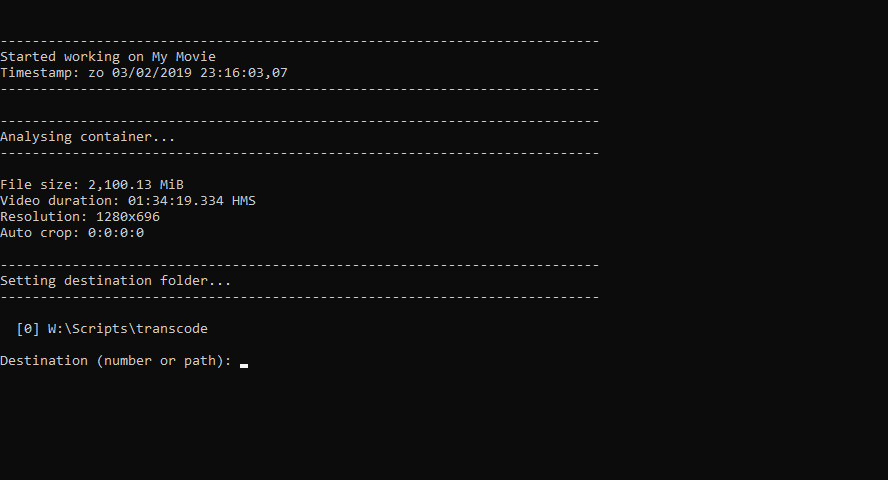There is only one destination path set, so I press “0”. I can also fill in a new destination path. This new destination path will then be saved in transcode_settings.ini and will be available next time under option . Now I have the option to remove some audio and subtitle tracks.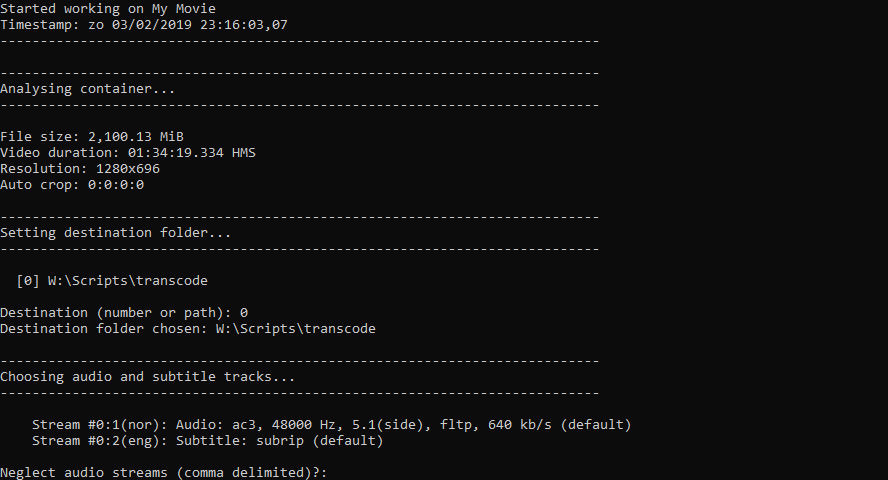Since I don’t want to remove any audio tracks I press “Enter”. Then I press “1” to remove the English subtitle stream.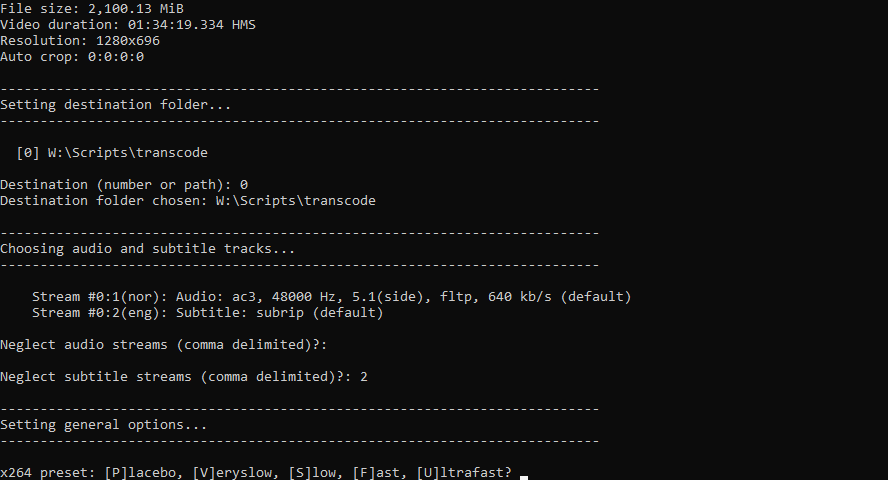Now I have to set some general transcoding options. I want a very good quality to bitrate ratio, so I select the very slow option by pressing “V”. I further select “A” for auto cropping (which is in this case equivalent to no cropping), and I also type “N” to signify that the movie is not black & white. Since I want a fixed size encode, I type “Y”, “N”, “N” to get an impression of what the optimal size would be.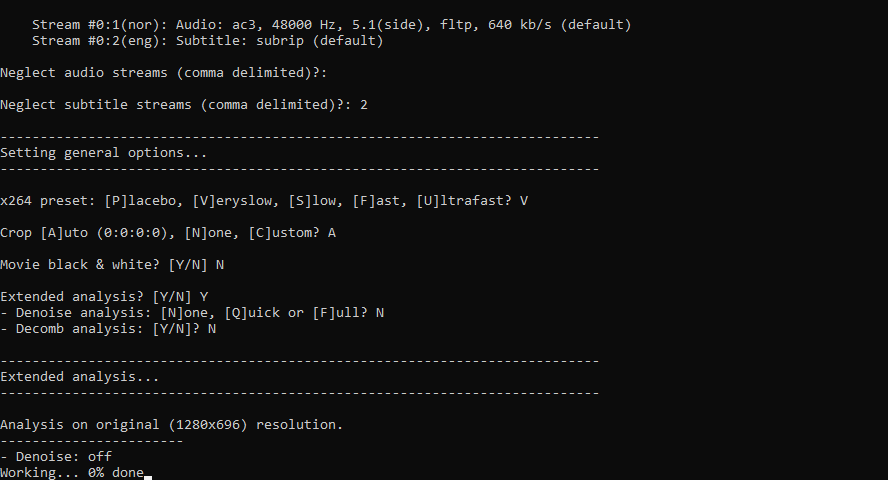The batch will now transcode a few samples based on the selected settings, and suggest a few optimal transcoding techniques: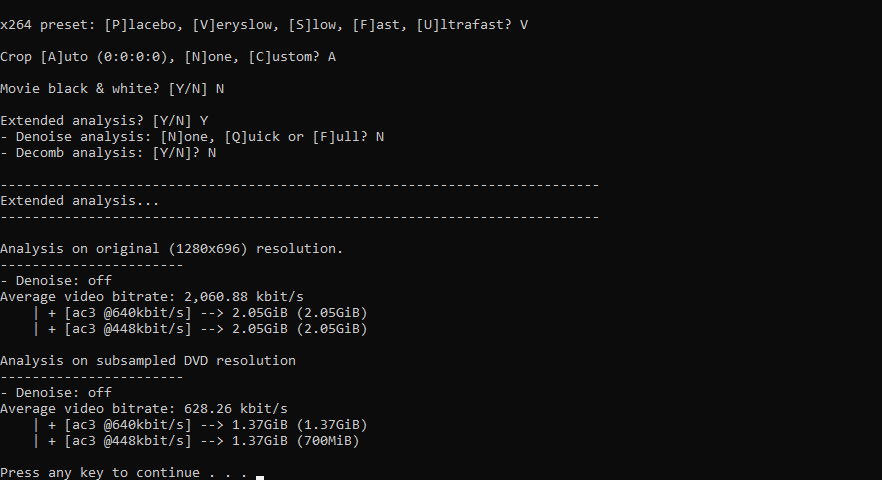For DVD resolution, if I transcode the audio stream to ac3 @448kbit/s, then the 700MiB size is 10% within the optimal range of predefined RF 20. Now I can select the handbrake transcode setting. I type “S” for a fixed size 2-pass transcode, “D” for AC3 448kbit/s audio transcode, “D” for DVD resolution, and twice “N” since I don’t want any noise reduction or decomb filter. Since I selected “S” for Size in the first option, I am now aked what my target size is. I type “1” for 700MiB and the transcoding process starts.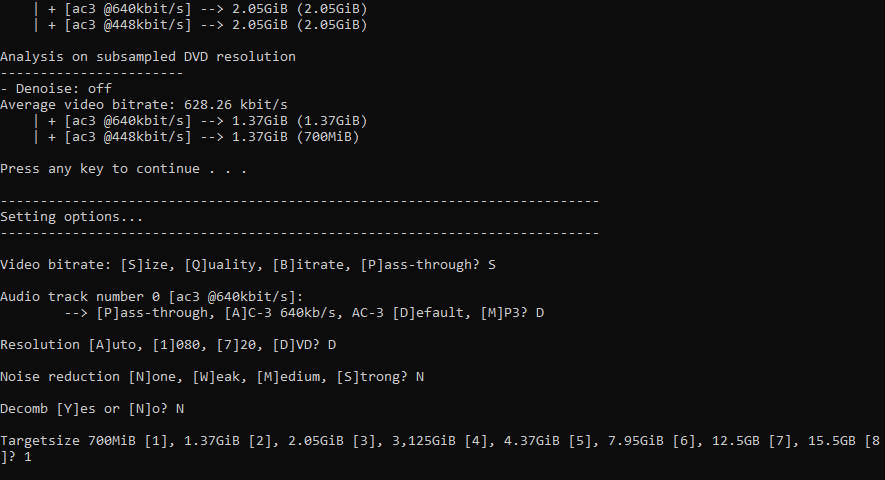Since this is an ordinary batch file, all the options are easily adjustable to one’s own needs.

Older Posts »